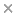BaseSpace
Correlation Engine 2.0Search sequence regions

###### filter terms:
Sizes of these terms reflect their relevance to your search.

Electrochemical behavior of homogentisic acid (HGA) has been studied in both aqueous and mixed solvent solution of water-acetonitrile. Physicochemical parameters of the electrochemical reaction of HGA in these solutions are obtained experimentally by cyclic voltammetry method and are also calculated theoretically using accurate ab initio calculations (G3MP2//B3LYP). Solvation energies are calculated using the available solvation model of CPCM. The pH dependence of the redox activity of HGA in aqueous and the mixture solutions at different temperatures was used for the experimental determination of the standard reduction potential and changes of entropy, enthalpy, and Gibbs free energy for the studied reaction. The experimental standard redox potential of the compound in aqueous solution was obtained to be 0.636 V versus the standard hydrogen electrode. There is a good agreement between the theoretical and experimental values (0.702 and 0.636 V) for the standard electrode potential of HGA. The changes of thermodynamic functions of solvation are also calculated from the differences between the solution-phase experimental values and the gas-phase theoretical values. Finally, using the value of solvation energy of HGA in water and acetonitrile solvents which calculated by the CPCM model of energy, we proposed an equation for calculating the standard redox potential of HGA in mixture solution of water and acetonitrile. A good agreement between the result of electrode potential calculated by the proposed equation and the experimental value confirms the validity of the theoretical models used here and the accuracy of experimental methods.

### Citation

Marzieh Eslami, Mansoor Namazian, Hamid R Zare. Electrooxidation of homogentisic acid in aqueous and mixed solvent solutions: experimental and theoretical studies. The journal of physical chemistry. B. 2013 Mar 7;117(9):2757-63

PMID: 23384055

View Full Text